Home Practice
For learners and parents For teachers and schools
Textbooks
Full catalogue
Pricing SupportLog in

We think you are located in United States. Is this correct?

# 8.3 Summary

## 8.3 Summary (EMBJC)

• Arc An arc is a portion of the circumference of a circle.
• Chord - a straight line joining the ends of an arc.
• Circumference - perimeter or boundary line of a circle.
• Radius ($$r$$) - any straight line from the centre of the circle to a point on the circumference.
• Diameter - a special chord that passes through the centre of the circle. A diameter is the length of a straight line segment from one point on the circumference to another point on the circumference, that passes through the centre of the circle.
• Segment A segment is a part of the circle that is cut off by a chord. A chord divides a circle into two segments.
• Tangent - a straight line that makes contact with a circle at only one point on the circumference.
• A tangent line is perpendicular to the radius, drawn at the point of contact with the circle.

temp textIf $$O$$ is the centre and $$OM \perp AB$$, then $$AM = MB$$. If $$O$$ is the centre and $$AM = MB$$, then $$A\hat{M}O = B\hat{M}O = \text{90}\text{°}$$. If $$AM = MB$$ and $$OM \perp AB$$, then $$\Rightarrow MO$$ passes through centre $$O$$.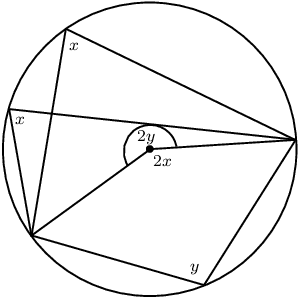If an arc subtends an angle at the centre of a circle and at the circumference, then the angle at the centre is twice the size of the angle at the circumference.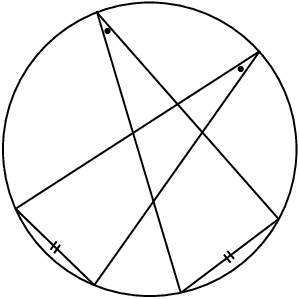Angles at the circumference subtended by the same arc (or arcs of equal length) are equal.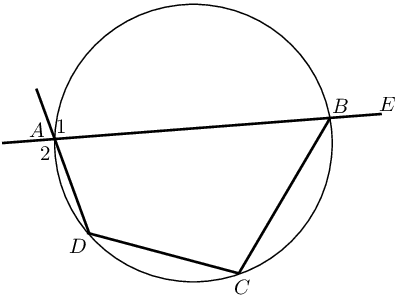The four sides of a cyclic quadrilateral $$ABCD$$ are chords of the circle with centre $$O$$. $$\hat{A} + \hat{C} = \text{180}\text{°}$$ (opp. $$\angle$$s supp.) $$\hat{B} + \hat{D} = \text{180}\text{°}$$ (opp. $$\angle$$s supp.) $$E\hat{B}C = \hat{D}$$ (ext. $$\angle$$ cyclic quad.) $$\hat{A}_1 = \hat{A}_2 = \hat{C}$$ (vert. opp. $$\angle$$, ext. $$\angle$$ cyclic quad.)Proving a quadrilateral is cyclic: If $$\hat{A} + \hat{C} = \text{180}\text{°}$$ or $$\hat{B} + \hat{D} = \text{180}\text{°}$$, then $$ABCD$$ is a cyclic quadrilateral.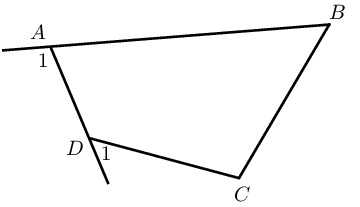If $$\hat{A}_1 = \hat{C}$$ or $$\hat{D}_1 = \hat{B}$$, then $$ABCD$$ is a cyclic quadrilateral.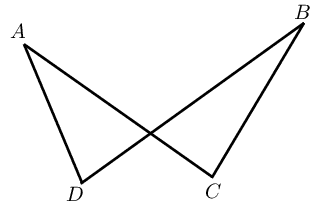If $$\hat{A} = \hat{B}$$ or $$\hat{C} = \hat{D}$$, then $$ABCD$$ is a cyclic quadrilateral.If $$AT$$ and $$BT$$ are tangents to circle $$O$$, then $$OA \perp AT$$ (tangent $$\perp$$ radius) $$OB \perp BT$$ (tangent $$\perp$$ radius) $$TA = TB$$ (tangents from same point equal)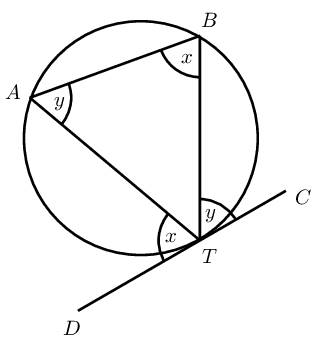If $$DC$$ is a tangent, then $$D\hat{T}A = T\hat{B}A$$ and $$C\hat{T}B = T\hat{A}B$$ If $$D\hat{T}A = T\hat{B}A$$ or $$C\hat{T}B = T\hat{A}B$$, then $$DC$$ is a tangent touching at $$T$$